# Kraken Daily Market Report for August 13 2020

## Overview

• Total trading volume at $433.5 million, which is significantly higher than the 30-day average of$264.9 million
• The above average volume was not driven by Bitcoin (which was 34% of the crypto trading volume). The high volume was a result of some exceptional gains from Algorand (+26%), WAVES (+56%), StorJ (+22%), and Gnosis (+30%).
• Ethereum also had a strong day (+9.6%), most of the gains were realized in a one hour span late in the day.

August 13, 2020
$433.5M traded across all markets today Crypto, EUR, USD, JPY, CAD, GBP, CHF, AUD XBT$11777.
↑1.8%
$157.4M ETH$424.50
↑9.6%
$119.0M LINK$17.143
↑4.1%
$61.6M USDT$1.0011
↑0.1%
$26.5M XTZ$4.1295
↓5.5%
$16.1M XRP$0.2948
↑4.4%
$14.5M ADA$0.1392
↑1.9%
$12.3M ALGO$0.6612
↑26%
$8.75M WAVES$3.3916
↑56%
$8.01M ATOM$6.0705
↑2.8%
$4.94M USDC$1.0001
↑0.03%
$4.31M LTC$57.065
↑4.7%
$4.22M BCH$295.69
↑3.9%
$3.82M COMP$204.54
↓6.3%
$3.75M KAVA$4.1891
↓6.8%
$2.1M ZEC$87.404
↑4.6%
$2.07M KNC$1.7361
↑3.2%
$1.56M STORJ$0.2743
↑22%
$1.53M EOS$3.1327
↑3.6%
$1.22M XLM$0.1009
↓0.7%
$1.18M XMR$91.970
↑2.6%
$1.16M ICX$0.4872
↑7.8%
$1.15M TRX$0.0223
↑11%
$1.13M REP$20.848
↑0.6%
$1.08M OMG$1.8365
↑8.3%
$1.03M LSK$1.6751
↑12%
$862K PAXG$1970.3
↑1.7%
$720K OXT$0.2019
↓1.9%
$656K DASH$92.601
↑0.9%
$619K ETC$6.9105
↑2.3%
$601K DAI$1.0174
↑0.12%
$543K REPV2$20.803
↑2.6%
$516K GNO$40.944
↑30%
$513K XDG$0.0034
↑0.6%
$504K BAT$0.2684
↓1.1%
$414K NANO$1.1517
↑2.5%
$387K QTUM$2.8562
↑2.1%
$346K MLN$25.576
↑0.9%
$331K SC$0.0033
↑1.7%
\$226K

#####################. Trading Volume by Asset. ##########################################

The figures below break down the trading volume of the largest, mid-size, and smallest assets. Cryptos are in purple, fiats are in blue. For each asset, the chart contains the daily trading volume in USD, and the percentage of the total trading volume. The percentages for fiats and cryptos are treated separately, so that they both add up to 100%.

Figure 1: Largest trading assets: trading volume (measured in USD) and its percentage of the total trading volume (August 13 2020)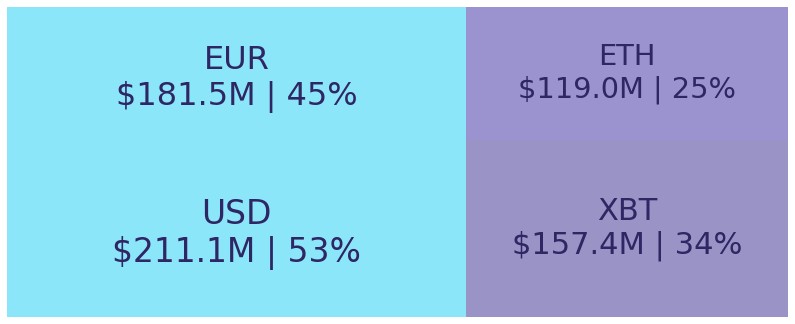Figure 2: Mid-size trading assets: (measured in USD) (August 13 2020)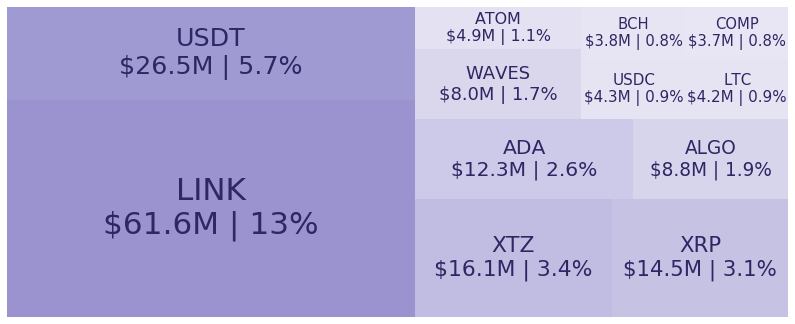Figure 3: Smallest trading assets: (measured in USD) (August 13 2020)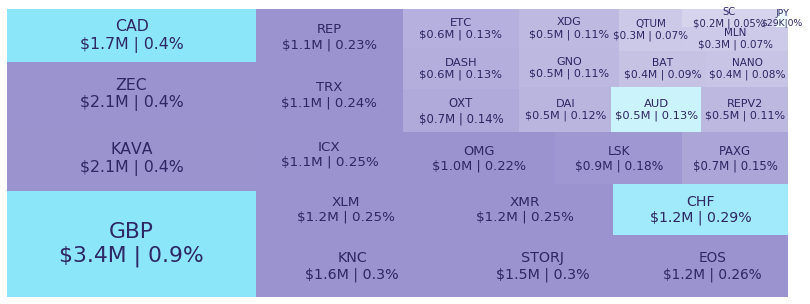Spread percentage is the width of the bid/ask spread divided by the bid/ask midpoint. The values are generated by taking the median spread percentage over each minute, then the average of the medians over the day.

Figure 4: Average spread % by pair (August 13 2020)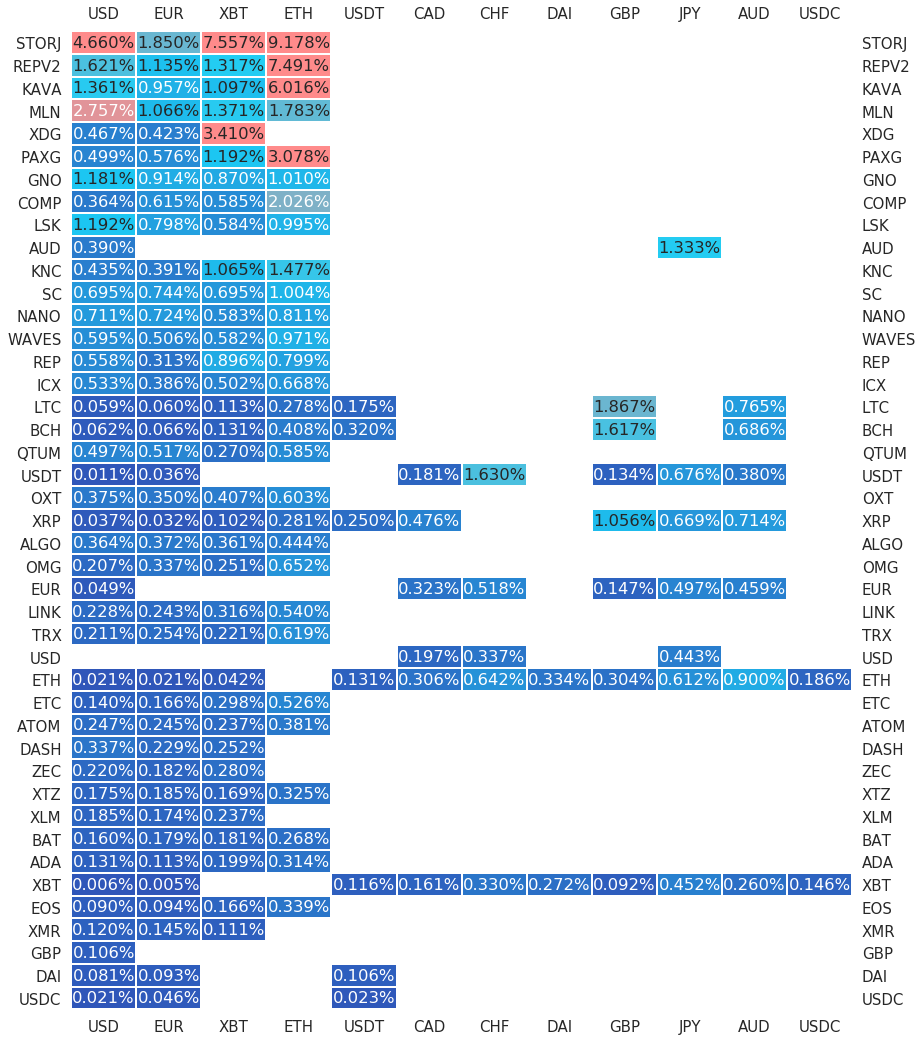.

#########. Returns and Volume ############################################

## Returns and Volume

Figure 5: Returns of the four highest volume pairs (August 13 2020)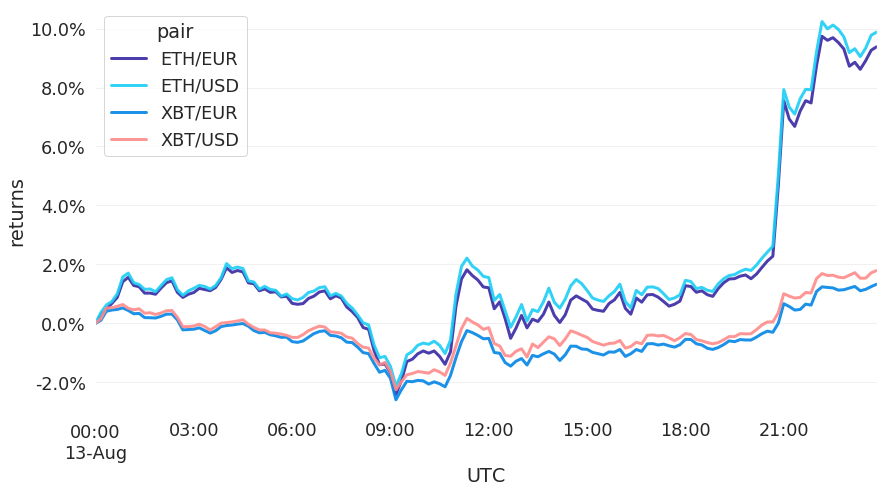Figure 6: Volume of the major currencies and an average line that fits the data to a sinusoidal curve to show the daily volume highs and lows (August 13 2020)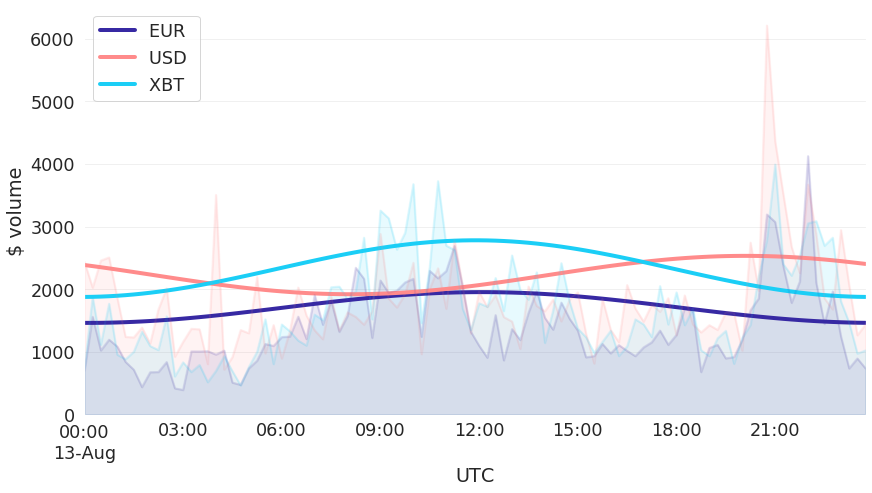###########. Daily Returns. #################################################

## Daily Returns %

Figure 7: Returns over USD and XBT. Relative volume and return size is indicated by the size of the font. (August 13 2020)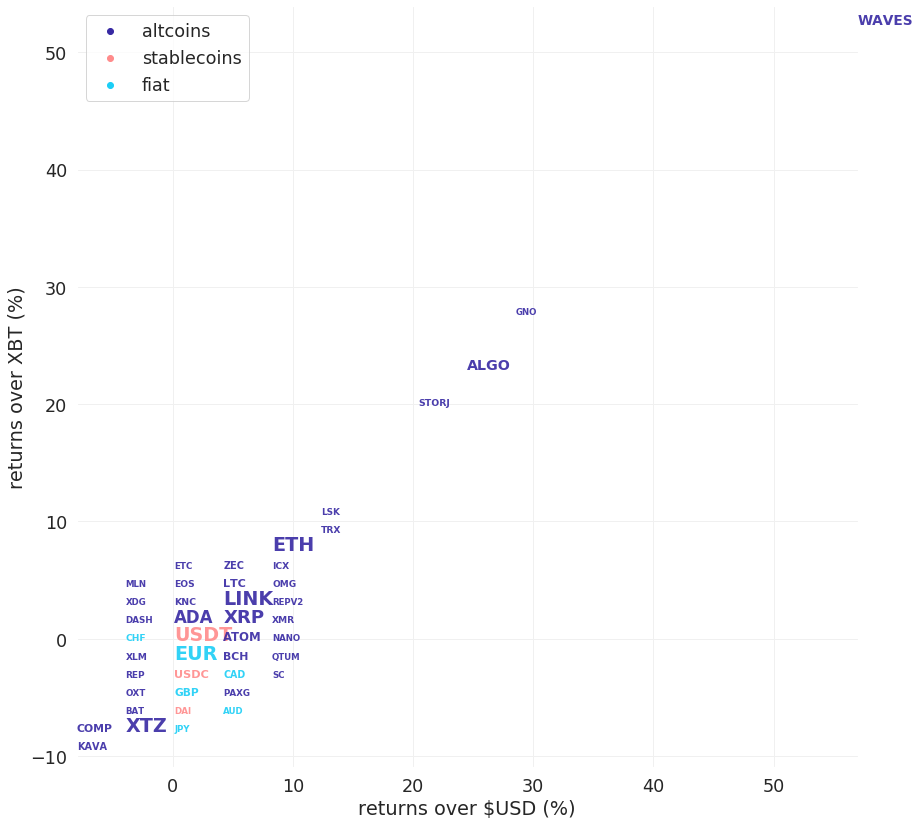###########. Disclaimer #################################################

The values generated in this report are from public market data distributed from Kraken WebSockets api. The total volumes and returns are calculated over the reporting day using UTC time.